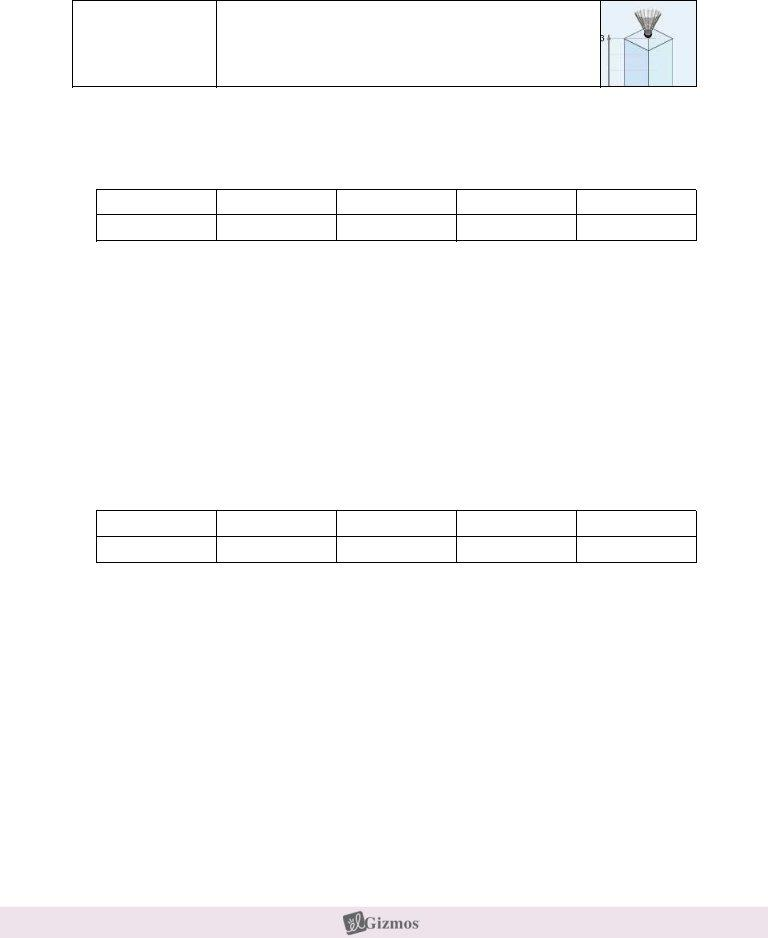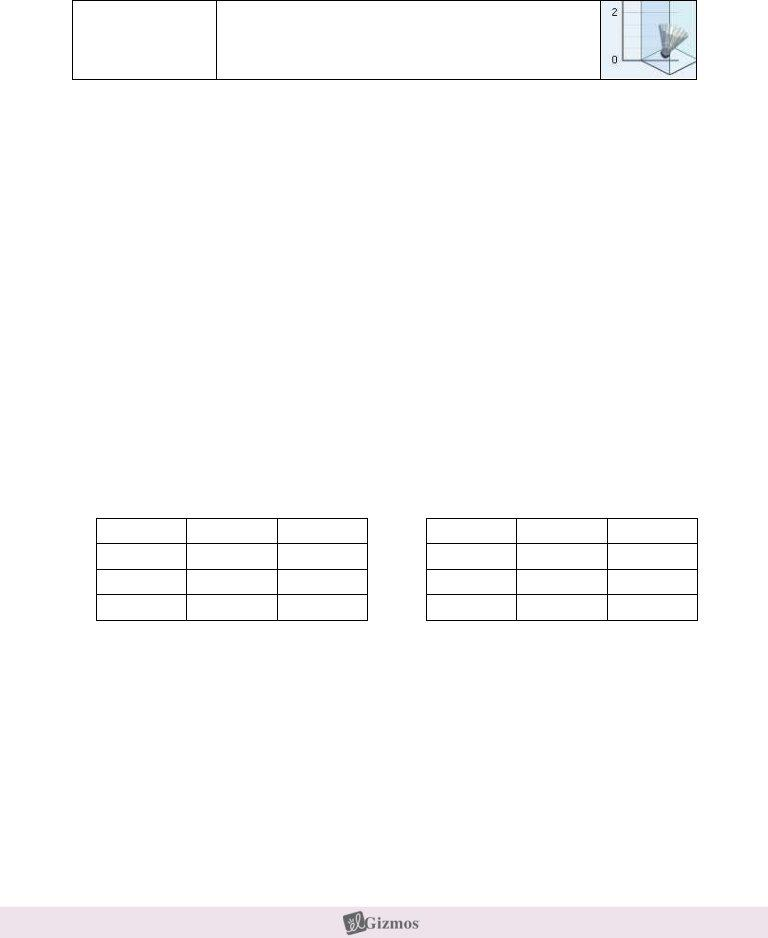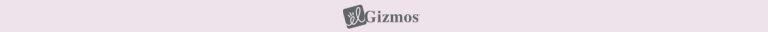## Student Exploration Laboratory Answers PDF Details

The Student Exploration Laboratory Answers Form is a fillable form that students can use to record their lab observations and findings. The form includes sections for recording the date, title of the experiment, introduction, materials list, procedure, results, and conclusions. The form can be customized to fit the needs of your specific lab experiments.

You will find info about the type of form you intend to fill out in the table. It can tell you the time it takes to fill out student exploration laboratory answers, what parts you will need to fill in and some further specific facts.

Form NameStudent Exploration Laboratory Answers
Form Length5 pages
Fillable?No
Fillable fields0
Avg. time to fill out1 min 15 sec
Other namesgizmo fall answer key, fall laboratory gizmo answers pdf, student exploration fall laboratory answer key, fall gizmo answer key pdf

## Form Preview ExampleName: ______________________________________ Date: ________________________

Student Exploration: Free-Fall Laboratory

Vocabulary: acceleration, air resistance, free fall, terminal velocity, velocity, vacuum

Prior Knowledge Questions (Do these BEFORE using the Gizmo.)

1.Suppose you dropped a feather and a hammer at the same time. Which would hit the ground first? _______________________________________________________________

2.Imagine repeating the experiment in an airless tube (vacuum). Would this change the result? Explain. ____________________________________________________________

_________________________________________________________________________

Gizmo Warm-up

The Free-Fall Laboratory Gizmoª allows you to measure the motion of an object in free fall. On the CONTROLS pane check that the Shuttlecock is selected, the Initial height is 3 meters, and the Atmosphere is Air.

1.Click Play () to release the shuttlecock. How long does it take to fall to the bottom? _________________________________________

2.Select the GRAPH tab. The box labeled H (m) should be checked, displaying a graph of height vs. time. What does this graph show?

_______________________________________________________

3.Turn on the V (m/s) box to see a graph of velocity vs. time. Velocity is the speed and direction of the object. Because the object is falling downward, its velocity is negative.

Does the velocity stay constant as the object drops? _____________

4.Turn on the A (m/s/s) box to see a graph of acceleration vs. time. Acceleration is the rate at which the velocity changes over time. What does this graph show?

_________________________________________________________________________Activity A: Falling objects

Get the Gizmo ready:

Click Reset ().

Select the CONTROLS tab.

Question: What factors affect how quickly an object falls?

1.Observe: Drop each item through Air from a height of 3 meters. Record how long it takes to

fall below. For the tennis ball, try to click Pause () when it hits the ground.

Shuttlecock

Cotton ball

Tennis ball

Rock

Pebble

2.Form a hypothesis: Why do some objects fall faster than others? _____________________

_________________________________________________________________________

3.Predict: A vacuum has no air. How do you think the results will change if the objects fall through a vacuum?

_________________________________________________________________________

4.Experiment: On the Atmosphere menu, select None. Drop each item again, and record the results below.

Shuttlecock

Cotton ball

Tennis ball

Rock

Pebble

5.Analyze: What happened when objects fell through a vacuum? _______________________

_________________________________________________________________________

6.Draw conclusions: Objects falling through air are slowed by the force of air resistance. Which objects were slowed the most by air resistance? Why do you think this is so?

_________________________________________________________________________

_________________________________________________________________________

_________________________________________________________________________

_________________________________________________________________________Activity B:

Terminal velocity

Get the Gizmo ready:

Click Reset.

Set the Initial height to 12 meters.

Set the Atmosphere to Air.

Question: How does air resistance affect falling objects?

1.Observe: Select the Shuttlecock. Choose the BAR CHART tab, and click Play. What do you notice about the velocity and acceleration of the shuttlecock?

_________________________________________________________________________

_________________________________________________________________________

When objects fall through air for a long time, they will eventually stop accelerating. Their velocity at this point is called terminal velocity.

2.Form hypothesis: How will an objectÕs size and mass affect its terminal velocity?

_________________________________________________________________________

3.Experiment: Click Reset. On the CONTROLS tab, select Manual settings. Set the height to 100 meters and the air density () to 1.3 kg/m3, close to actual air density at sea level.

For each combination of mass and radius in the charts below, find the terminal velocity

(VTERMINAL) of the object. Use the BAR CHART tab to find the terminal velocity. (Hint: Turn on Show numerical values.)

Mass

1.0g 10.0 g 50.0 g

3.0cm

3.0cm

3.0cm

VTERMINAL

Mass

10.0g

10.0g

10.0g

2.0cm

5.0cm

10.0 cm

VTERMINAL

4.Analyze: Your data show how mass and radius affect terminal velocity.

A.What was the effect of increasing mass? __________________________________

B.What was the effect of increasing radius? __________________________________

5.Apply: If you wanted to use a device to slow your fall, what properties should it have?

_________________________________________________________________________Activity C:

Acceleration, distance, and time

Get the Gizmo ready:

Click Reset.

Select Common objects.

Set the Atmosphere to None.

Question: How long does it take an object to fall from a given height?

1.Observe: Select the Rock, and set the Initial height to 3 meters. Choose the GRAPH tab, and click Play to drop the rock through a vacuum. Turn on all three graphs.

A.What is the shape of the graph of velocity vs. time? __________________________

B.What is the shape of the graph of acceleration vs. time? ______________________

2.Analyze: Select the TABLE tab and look at the V (m/s) column.

A.The starting velocity was 0 m/s, and the final velocity was -7.68 m/s. Based on this, what was the average velocity of the rock? _________________________________

B.In general, how do you find the average velocity of any object falling in a vacuum? (Assume you know the final velocity.) _____________________________________

3.Calculate: Distance, average velocity, and time are related by the equation, d = vaverage ¥ t

A.How much time did it take the rock to fall? _________________________________

B.What is the product of the average velocity and time? ________________________

C.Does this equal the distance that the rock fell? ______________________________

4.Calculate: The acceleration of any object due to EarthÕs gravity is -9.81 m/s2. For every second an object falls, its velocity changes by 9.81 meters per second. For several different times on the table, multiply the time by the acceleration.

A.What do you notice? __________________________________________________

B.If you know the acceleration and time, how can you calculate the final velocity?

___________________________________________________________________

C.Challenge: If you know the acceleration and time, how can you calculate the average

velocity? _____________________________________________________

(Activity C continued on next page)Activity C (continued from previous page)

5.Make a rule: So far you have figured out two rules that relate time, acceleration, average velocity, and distance. Review these rules now.

A.How do you find average velocity (vaverage) from acceleration (a) and time (t)?

___________________________________________________________________

B.How do you find distance (d) from average velocity (vaverage) and time (t)?

___________________________________________________________________

C.Now put the two equations together. Substitute your result in equation A for the

(vaverage) term in equation B. Your final equation should only have d, a, and t terms.

___________________________________________________________________

6.Apply: Use your rule to solve the following problems. Check your answers with the Gizmo. Assume that each fall takes place in a vacuum with an acceleration of -9.81 m/s2.

A.A rock falls for 1.43 seconds. How far did it fall? _____________________________

B.How long will it take for a rock to fall 12 meters? _____________________________

C.A rock falls for 4 seconds. How far did it fall? _______________________________

D.A rock falls for 3 seconds. What was its velocity when it hit the ground? __________

E.How long will it take for a rock to fall 50 meters? _____________________________

## Watch Student Exploration Laboratory Answers Video Instruction

If you believe this page is infringing on your copyright, please familiarize yourself with and follow our DMCA notice and takedown process - click here to proceed .